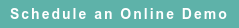# RoyaltyStat Blog

### Posts by Topic

Zero Intercept Linear Profit Function

The typical OECD TNMM (CPM in the U.S.) prescribes a linear statistical function to test the arm's length character of “net” profits (Y) in terms of the net sales (X):

(1)     Yi = α Xi considering i = 1, 2, …, N comparables

where α is the estimated “net” profit margin. For simplification, we set aside a random error term that is added to equation (1). The controlled taxpayer ("tested party") is the case N + 1.

Non-Linear Profit Function

Instead of equation (1), "net" profits and sales may be represented by a power function:

(2)     Yi = α Xiβ

Power functions are pervasive in economic estimates. Equation (2) states that Yi is proportional to Xiβ . In this case, the profit margin is the slope coefficient of equation (2), which below we show is different from α. A power function is appropriate e.g. when the selection of comparables to the tested party includes small and large companies or when the residual variance is not constant.

Slope Coefficient

The slope coefficient of equation (2) is the derivative of Y with respect to X:

(3)     Yi’ = α β Xi β – 1

where Yi’ (variable followed by an apostrophe) is the first derivative. We use two rules of exponents (1/X = X1) and (Xm/Xn = Xmn) to obtain a clearer expression for the slope coefficient of the power function:

(4)     Yi’ = (α β Xi β / Xi) = β (Yi / Xi)

In equation (4), the regression coefficient (β) is multiplied by a selected comparable profit margin (Yi / Xi). It’s sensible to use the comparable median or the average (free of outliers) profit margin of the selected comparables as a multiplicative constant of β, and thus determine the arm’s length profit margin of the “tested party”. In this case, a scatter plot of Yi versus Xi can reveal if the power function is a better (more reliable) fit of the company comparable data than the linear prescription of the OECD.

It’s easy to compute the slope = β (Yi / Xi) using Excel. Starting with the Y and X columns, we can create two additional columns using the Excel natural logarithms function LN(Y) and LN(X). Next, we use the Excel function SLOPE (LN(Y), LN(X)) = β. As a final step, we multiply the computed slope by the median or the average (Y/X) in order to obtain β (Yi / Xi). To compute the regression coefficients (intercept and slope) together with their standard errors, we can use instead the Excel function LINEST ( ). We think that it’s better to learn a dedicated statistical package such as Minitab, Systat, or SPSS (instead of Excel) because we can diagnose more effectively the regression residuals and ascertain the most reliable estimate of the arm's length profit margin. In RoyaltyStat, we have built-in regression functions to estimate the profit margin using the linear, power, exponential, and other statistical curves.

Published on May 24, 2016 5:18:06 AM

Ednaldo Silva (Ph.D.) is founder and managing director of RoyaltyStat. He helped draft the US transfer pricing regulations and developed the comparable profits method called TNNM by the OECD. He can be contacted at: esilva@royaltystat.com

RoyaltyStat provides premier online databases of royalty rates extracted from unredacted license agreements
and normalized company financials (income statement, balance sheet, cash flow). We provide high-quality data, built-in analytical tools, customer training and attentive technical support.Topics: Net Profit Indicator